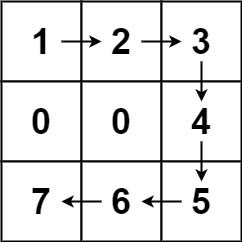675. Cut Off Trees for Golf Event

You are asked to cut off all the trees in a forest for a golf event. The forest is represented as an `m x n` matrix. In this matrix:

• `0` means the cell cannot be walked through.
• `1` represents an empty cell that can be walked through.
• A number greater than `1` represents a tree in a cell that can be walked through, and this number is the tree's height.

In one step, you can walk in any of the four directions: north, east, south, and west. If you are standing in a cell with a tree, you can choose whether to cut it off.

You must cut off the trees in order from shortest to tallest. When you cut off a tree, the value at its cell becomes `1` (an empty cell).

Starting from the point `(0, 0)`, return the minimum steps you need to walk to cut off all the trees. If you cannot cut off all the trees, return `-1`.

You are guaranteed that no two trees have the same height, and there is at least one tree needs to be cut off.

Example 1:```Input: forest = [[1,2,3],[0,0,4],[7,6,5]]
Output: 6
Explanation: Following the path above allows you to cut off the trees from shortest to tallest in 6 steps.
```

Example 2:```Input: forest = [[1,2,3],[0,0,0],[7,6,5]]
Output: -1
Explanation: The trees in the bottom row cannot be accessed as the middle row is blocked.
```

Example 3:

```Input: forest = [[2,3,4],[0,0,5],[8,7,6]]
Output: 6
Explanation: You can follow the same path as Example 1 to cut off all the trees.
Note that you can cut off the first tree at (0, 0) before making any steps.
```

Constraints:

• `m == forest.length`
• `n == forest[i].length`
• `1 <= m, n <= 50`
• `0 <= forest[i][j] <= 109`

675. Cut Off Trees for Golf Event
``````struct Solution;

use std::cmp::Reverse;
use std::collections::BinaryHeap;
use std::collections::VecDeque;

impl Solution {
fn cut_off_tree(forest: Vec<Vec<i32>>) -> i32 {
let n = forest.len();
let m = forest.len();
let mut queue: BinaryHeap<(Reverse<i32>, usize, usize)> = BinaryHeap::new();
for i in 0..n {
for j in 0..m {
if forest[i][j] > 1 {
queue.push((Reverse(forest[i][j]), i, j));
}
}
}
let mut i = 0;
let mut j = 0;
let mut res = 0;
while let Some((_, r, c)) = queue.pop() {
let d = Self::bfs(i, j, r, c, &forest, n, m);
if d < 0 {
return -1;
}
i = r;
j = c;
res += d;
}
res
}

fn bfs(i: usize, j: usize, r: usize, c: usize, forest: &[Vec<i32>], n: usize, m: usize) -> i32 {
let mut queue: VecDeque<(usize, usize, i32)> = VecDeque::new();
let mut visited: Vec<Vec<bool>> = vec![vec![false; m]; n];
queue.push_back((i, j, 0));
visited[i][j] = true;
while let Some((i, j, d)) = queue.pop_front() {
if r == i && c == j {
return d;
}
if i > 0 && forest[i - 1][j] > 0 && !visited[i - 1][j] {
visited[i - 1][j] = true;
queue.push_back((i - 1, j, d + 1));
}
if j > 0 && forest[i][j - 1] > 0 && !visited[i][j - 1] {
visited[i][j - 1] = true;
queue.push_back((i, j - 1, d + 1));
}
if i + 1 < n && forest[i + 1][j] > 0 && !visited[i + 1][j] {
visited[i + 1][j] = true;
queue.push_back((i + 1, j, d + 1));
}
if j + 1 < m && forest[i][j + 1] > 0 && !visited[i][j + 1] {
visited[i][j + 1] = true;
queue.push_back((i, j + 1, d + 1));
}
}
-1
}
}

#[test]
fn test() {
let forest = vec_vec_i32![[1, 2, 3], [0, 0, 4], [7, 6, 5]];
let res = 6;
assert_eq!(Solution::cut_off_tree(forest), res);
let forest = vec_vec_i32![[1, 2, 3], [0, 0, 0], [7, 6, 5]];
let res = -1;
assert_eq!(Solution::cut_off_tree(forest), res);
let forest = vec_vec_i32![[2, 3, 4], [0, 0, 5], [8, 7, 6]];
let res = 6;
assert_eq!(Solution::cut_off_tree(forest), res);
}
``````